Home / Blog / Data Science / Understanding Exponential Trend Models for Time Series Forecasting

# Understanding Exponential Trend Models for Time Series Forecasting

• November 01, 2023
• 2277
• 58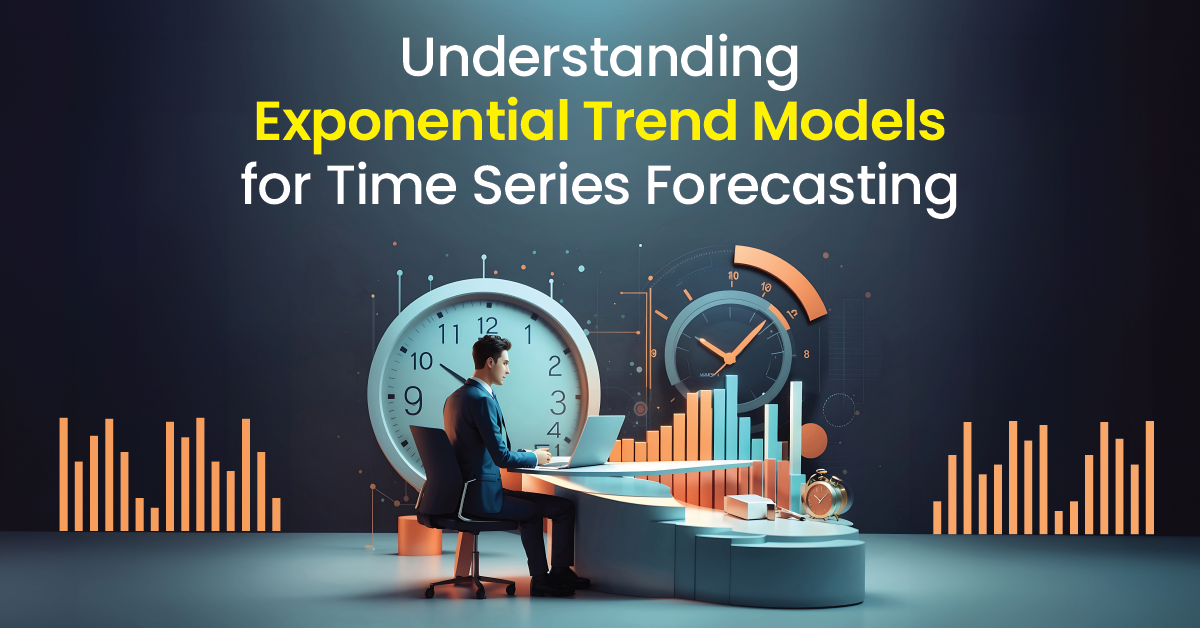### Meet the Author : Mr. Bharani Kumar

Bharani Kumar Depuru is a well known IT personality from Hyderabad. He is the Founder and Director of Innodatatics Pvt Ltd and 360DigiTMG. Bharani Kumar is an IIT and ISB alumni with more than 17 years of experience, he held prominent positions in the IT elites like HSBC, ITC Infotech, Infosys, and Deloitte. He is a prevalent IT consultant specializing in Industrial Revolution 4.0 implementation, Data Analytics practice setup, Artificial Intelligence, Big Data Analytics, Industrial IoT, Business Intelligence and Business Management. Bharani Kumar is also the chief trainer at 360DigiTMG with more than Ten years of experience and has been making the IT transition journey easy for his students. 360DigiTMG is at the forefront of delivering quality education, thereby bridging the gap between academia and industry.

## Introduction

Time series forecasting is a vital tool in various industries, allowing businesses to predict future trends based on historical data. It involves understanding and applying different trend models to make accurate projections. In this blog post, we will delve into the intricacies of exponential trend models, which provide significant insights into exponential growth or decay patterns in time series data.

Are you looking to become a Data Scientist? Go through 360DigiTMG's Data Science course training in Hyderabad!

## Basics of Exponential Trend Models

Exponential trend models, often used for time series forecasting, capture the exponential growth or decay present in the data. These models are applicable when the rate of change in the data is proportional to the current value. Mathematical equations drive these models, and the basic form of an exponential trend model is as follows: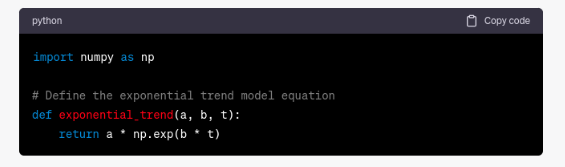Here, `a` represents the observed value, `t` denotes time, `a` influences the initial value or intercept, `b` determines the growth rate or decay, and ε represents the error term.

The components of this model are essential for understanding and accurately representing the underlying patterns in the data. The intercept (`a`) determines the starting point of the exponential growth or decay, while the growth rate (`b`) quantifies how fast the change occurs. The error term (`ε`) captures any random or unpredictable fluctuations that deviate from the underlying exponential trend.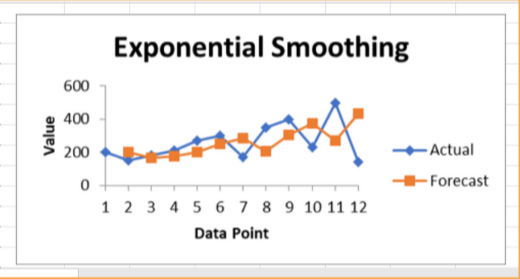To gain a better intuitive understanding, picture exponential growth as a snowball rolling down a hill, gradually gaining momentum and speed. Similarly, exponential decay can be visualized as a diminishing firework sparkler, fading away over time. These models encapsulate such behaviors, making them invaluable in analyzing time series data.

## Types of Exponential Trend Models

Exponential trend models come in various forms, each catering to specific forecasting needs. Let's explore three prominent types:### Single Exponential Smoothing

Single Exponential smoothing is an entry-level technique that allows for basic forecasting by assigning exponentially decreasing weights to historical data points. By considering only the most recent observations, this model places greater emphasis on immediate trends. It is particularly useful when there is no discernible trend or seasonality in the data.

Want to learn more about data science? Enroll in this Data Science course training in Bangalore to do so.

The formula for Single Exponential Smoothing is as follows:

Ft+1=α×Yt+(1−α)×FtFt+1​=α×Yt​+(1−α)×Ft​

Where:

Ft+1Ft+1​ is the forecast for the next period.

YtYt​ is the actual value in the current period.

FtFt​ is the forecast for the current period.

αα is the smoothing parameter (0 < αα < 1), which determines the weight given to the most recent observation.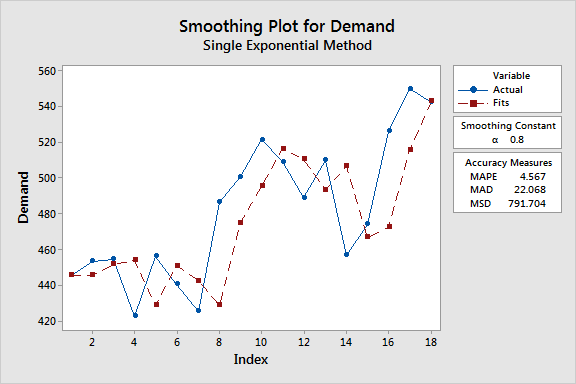Single Exponential Smoothing is easy to implement and provides forecasts that respond quickly to changes in the data. However, it may not perform well when dealing with data that has more complex patterns or seasonality.

## Double Exponential Smoothing (Holt's Linear Exponential Smoothing)

Double Exponential Smoothing, also known as Holt's Linear Exponential Smoothing, extends the single exponential smoothing model by incorporating a component for trend. This technique takes into account both the current level and trend when making forecasts. By considering the latest trend, this model provides better accuracy for time series data exhibiting a clear linear trend.

The formula for Double Exponential Smoothing is as follows:

Lt=α×Yt+(1−α)×(Lt−1+Tt−1)Lt​=α×Yt​+(1−α)×(Lt−1​+Tt−1​)

Tt=β×(Lt−Lt−1)+(1−β)×Tt−1Tt​=β×(Lt​−Lt−1​)+(1−β)×Tt−1​

Ft+1=Lt+TtFt+1​=Lt​+Tt​

Where:

LtLt​ represents the level component at time tt.

TtTt​ represents the trend component at time tt.

Ft+1Ft+1​ is the forecast for the next period.

αα and ββ are smoothing parameters (0 < αα, ββ < 1).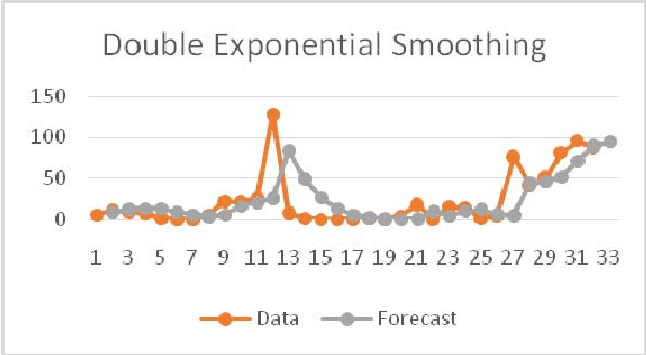Double Exponential Smoothing is useful for capturing data with both a trend and a level component, making it a valuable tool in business forecasting, such as sales or demand forecasting.

## Triple Exponential Smoothing (Holt-Winters Exponential Smoothing)

Triple Exponential Smoothing, or Holt-Winters Exponential Smoothing, goes a step further by incorporating seasonality in addition to trend. This model is especially useful for datasets that exhibit recurring patterns over specific intervals. By capturing both the level, trend, and seasonality components, it produces accurate forecasts for time series data with multiple seasonal patterns.

There are also specialized versions of exponential trend models available to cater to diverse scenarios. These include variants that handle dampened trend models or address specific data characteristics.

The formula for Triple Exponential Smoothing includes three equations for updating the level, trend, and seasonal components:

Lt=α×YtSt−m+(1−α)×(Lt−1+Tt−1)Lt​=α×St−m​Yt​​+(1−α)×(Lt−1​+Tt−1​)

Tt=β×(Lt−Lt−1)+(1−β)×Tt−1Tt​=β×(Lt​−Lt−1​)+(1−β)×Tt−1​

St=γ×YtLt+(1−γ)×St−mSt​=γ×Lt​Yt​​+(1−γ)×St−m​

Ft+m=(Lt+Tt)×St−m+1Ft+m​=(Lt​+Tt​)×St−m+1​

Where:

LtLt​ represents the level component at time tt.

TtTt​ represents the trend component at time tt.

StSt​ represents the seasonal component at time tt.

Ft+mFt+m​ is the forecast for mm periods ahead.

αα, ββ, and γγ are smoothing parameters (0 < αα, ββ, γγ < 1), with γγ controlling the seasonality.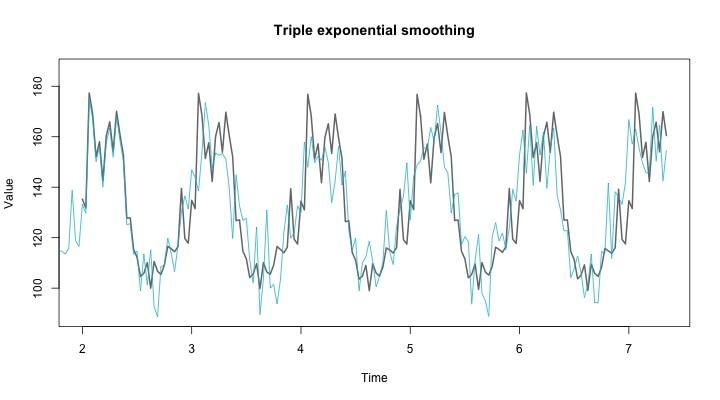Triple Exponential Smoothing is highly effective for forecasting data with complex patterns that exhibit seasonality. It is widely used in various industries, including retail, finance, and supply chain management.

## Implementing Exponential Trend Models in Python

Implementing exponential trend models in Python can be done using libraries like NumPy and pandas. Let's explore the process step by step:

1. Start by importing the necessary libraries, including NumPy and pandas.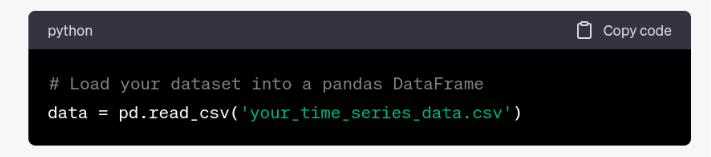3. Choose the appropriate exponential trend model based on the characteristics of your data.

4. Fit the model to the data using techniques like least squares regression.

Earn yourself a promising career in Data Science by enrolling in the in Data Science Course training in Chennai offered by 360DigiTMG.

5. Visualize the fitted models and residuals to assess the goodness of fit and identify any anomalies.

By following these steps, you can effortlessly implement each type of exponential trend model and gain valuable insights into your time series data.

## Forecasting with Exponential Trend Models

Forecasting with exponential trend models involves making predictions about future values based on the fitted models. These models utilize the captured patterns to project the future growth or decay. To perform accurate forecasting, consider the following steps:

1. Utilize the fitted exponential trend models obtained from the previous steps.

2. Adjust the models to account for the desired forecasting horizon.

3. Use the adjusted models to estimate values for the future time steps.

4. Evaluate the reliability and accuracy of the forecasts based on validation techniques and performance metrics.

By understanding the limitations and assumptions associated with exponential trend models, you can ensure more accurate and reliable forecasts.

## Model Evaluation and Performance Metrics

Evaluating the performance of exponential trend models is crucial in determining the accuracy of your predictions. Key performance metrics include Mean Squared Error (MSE) and Root Mean Squared Error (RMSE). These metrics provide insights into the average squared differences between the predicted and actual values, with lower values indicating better accuracy. Implement the following steps to assess model performance:

1. Calculate the MSE and RMSE using appropriate Python code.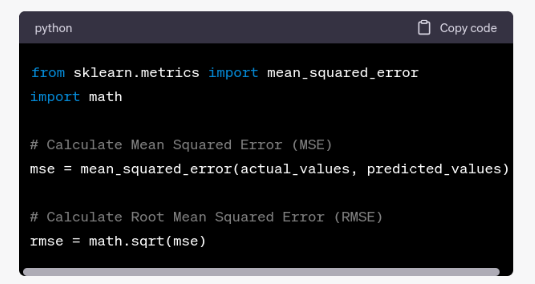2. Interpret the resulting metrics by considering their absolute values and comparing them to other models or benchmarks.

3. Refine your models or forecasting techniques based on the performance evaluation.

By regularly evaluating the performance of your exponential trend models, you can continuously improve the accuracy of your forecasts.

## Choosing the Right Exponential Trend ModelSelecting the most suitable exponential trend model for your specific forecasting needs requires careful considerations. Factors to consider include the nature of your data, the presence or absence of trends or seasonality, and the desired complexity of the model. To make an informed decision, follow these guidelines:

1. Assess the characteristics of your dataset: Begin by thoroughly examining your time series data. Identify whether there is a clear trend, seasonality, or both. Understanding these inherent patterns in your data is crucial for selecting the appropriate model. Single Exponential Smoothing may suffice for data with a straightforward trend, while data with both trend and seasonality may require the advanced Triple Exponential Smoothing method.

2. Determine the importance of capturing trend and seasonality accurately: Consider the implications of forecasting errors in your specific application. In some cases, accurately capturing the underlying trends and seasonality may be critical for decision-making, such as in demand forecasting or financial analysis. In other scenarios, a simpler model that provides a rough estimate may be sufficient.

3. Consider the level of complexity appropriate for your dataset and available computing resources:Complex models like Triple Exponential Smoothing require more computational power and may not be suitable for large datasets or real-time forecasting. Be realistic about your computational resources and time constraints when selecting a model. Sometimes, a less complex model with reasonable accuracy is preferable due to efficiency and ease of implementation.

4. Evaluate the performance of different exponential trend models using validation techniques: Before finalizing your choice, it's essential to evaluate the performance of various models on historical data. Use techniques like cross-validation or hold-out validation to assess how well each model performs in terms of accuracy and robustness. This step will provide you with empirical evidence to support your model selection.

Understanding these factors will assist you in choosing the optimal exponential trend model that aligns with the unique characteristics of your time series data. Remember that the choice of the right model is not a one-size-fits-all decision; it should be tailored to your specific forecasting goals, data, and constraints. Continuously monitoring and refining your chosen model's performance is also crucial to ensure its effectiveness as new data becomes available and your forecasting needs evolve.

## Conclusion

In conclusion, exponential trend models play a vital role in time series forecasting, providing insights into exponential growth or decay patterns. By understanding the basics of exponential trend models, exploring the various types, and implementing them in Python, you can make accurate predictions for your time series data.

Remember to evaluate the performance of your models using appropriate metrics and choose the right model based on your data characteristics. With these tools at your disposal, you can unlock the power of exponential trend models and make informed business decisions.

We hope this blog post has provided you with valuable insights into the world of exponential trend models. We encourage you to dive deeper and experiment with different time series datasets to further enhance your forecasting skills. Whether you are working in finance, marketing, or any other field that relies on data-driven decision-making, mastering exponential trend models can be a game-changer. By staying curious and continuously refining your forecasting techniques, you'll be well-equipped to tackle the challenges of predicting future trends and staying ahead in today's dynamic and competitive business environment.

### Data Analyst Courses in Other Locations